# Introduction To Continuity

Go back to  'LCD'

We start with a very intuitive introduction to continuity. Consider the two graphs given in the figure below: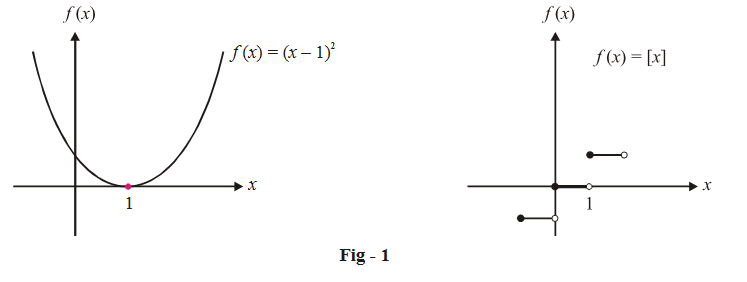Our purpose is to analyse the behaviour of these functions around the region x = 1.

The obvious visual difference between the two graphs around x = 1 is that whereas the first graph passes uninterrupted (without a break) through x = 1, the second function suffers a break at x = 1 (there is a jump).

This visual difference, put into mathematical language, gives us the concept and definition of continuity. Mathematically, we say that the function $$f\left( x \right) = {\left( {x - 1} \right)^2}$$  is continuous at x = 1 while  $$f\left( x \right) = \left[ x \right]$$ is discontinuous at x = 1

For   $$f\left( x \right) = {\left( {x - 1} \right)^2},$$

$$LHL \left( {{\rm{at}}\,x = 1} \right) = \mathop {\lim }\limits_{x \to {1^ - }} {\left( {x - 1} \right)^2} = 0$$

and

$$RHL \left( {{\rm{at}}\,x = 1} \right) = \mathop {\lim }\limits_{x \to {1^ + }} {\left( {x - 1} \right)^2} = 0$$

and

$$f(1) = 0$$

$$\Rightarrow LHL = RHL = f(1)$$

For             $$f\left( x \right) = \left[ x \right],$$

$${\rm{LHL }}\left( {{\rm{at\, }}x = 1} \right) = \mathop {\lim }\limits_{x \to {1^ - }} \left[ x \right] = 0$$

and

$${\rm{RHL }}\left( {{\rm{at\, }}x = 1} \right) = \mathop {\lim }\limits_{x \to {1^ + }} \left[ x \right] = 1$$

and

$$f(1) = 1$$

$$\Rightarrow \,\,\,\,\,{\rm{ }}\,{\rm{LHL}} \ne {\rm{RHL}}\,{\rm{ = }}\,\,f\left( 1 \right)$$

From the discussion above, try to see that for a function to be continuous at x = a,  all the three quantities, namely, LHL, RHL and f (a) should be equal. In any other scenario, the function becomes discontinuous.

Discontinuities therefore arise in the following cases:

(a)         One or more than one of the three quantities, LHL, RHL and f(a) is not defined . Lets consider some examples:

(i)     \begin{align}f\left( x \right) = \frac{1}{x}\end{align} around x = 0.

$${\rm{LHL}}\,{\rm{ = }}\, - \infty ,{\rm{ RHL}}\,{\rm{ = }}\, + \infty ,\,f\left( 0 \right)$$  is not defined. Therefore, \begin{align}f\left( x \right) = \frac{1}{x}\end{align}   is discontinuous at x = 0 which is obvious from the graph: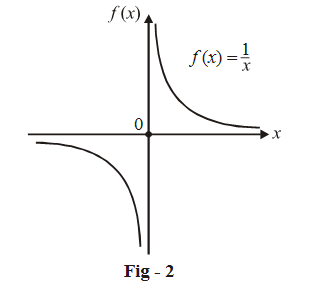(ii)   \begin{align}f\left( x \right) = \left\{ {\frac{{{x^2} - 1}}{{x - 1}}{\rm{\,for\, }}x \ne 1} \right\}\end{align} around x = 1

$$LHL{\rm{ }} = {\rm{ }}RHL{\rm{ }} = {\rm{ }}2$$ but $$f(1)$$ is not defined. Therefore, this function’s graph has a hole at x = 1; it is discontinuous at x = 1: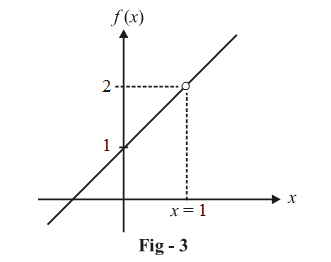(b)        All the three quantities are defined, but any pair of them is unequal (or all three are unequal). Lets go over some examples again:

(i)  $$f(x){\rm{ }} = {\rm{ }}[x] \text{around any integer }I$$

$$LHL{\rm{ }} = I-1,{\rm{ }}RHL{\rm{ }} = I,f\left( I \right){\rm{ \;}} = I$$

$$\Rightarrow {\rm{LHL}} \ne {\rm{RHL}}\,{\rm{ = }}\,f\left( I \right)$$ so this function is discontinuous at all integers as

(ii) $$f\left( x \right) = \left\{ x \right\}{\rm{ }}around{\rm{\; }}any{\rm{\; }}integer{\rm{\;}}I$$

$$LHL{\rm{ }} = 1,{\rm{ }}RHL{\rm{ }} = {\rm{ }}0{\rm{ }},f\left( I \right){\rm{ }} = {\rm{ }}0$$

$$\Rightarrow {\rm{LHL}} \ne {\rm{RHL}}\;{\rm{ = }}\;f\left( I \right)$$  so this function is also discontinuous at all integers.

(iii)        $$f\left( x \right) = \left\{ \begin{gathered}1,\,\,\,x \notin \mathbb{Z} \hfill \\0,\,\,\,x \in \mathbb{Z} \hfill \\\end{gathered} \right\}$$      around any integer I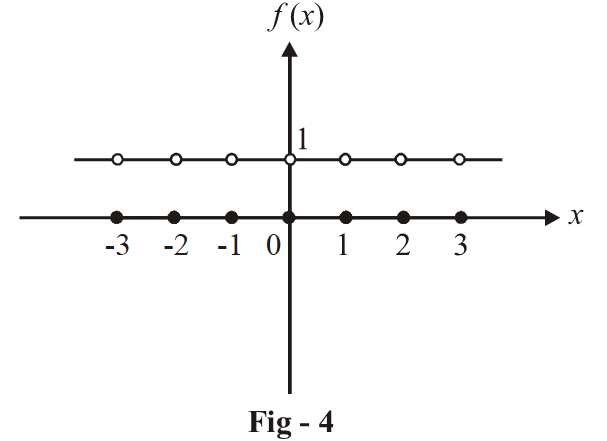From the figure, we notice that at any integer $$I,{\rm{ }}LHL{\rm{ }} = {\rm{ }}1,{\rm{ }}RHL{\rm{ }} = 1,f\left( I \right){\rm{ }} = {\rm{ }}0$$

$$\Rightarrow {\rm{LHL}}\;{\rm{ = }}\;{\rm{RHL}} \ne f\left( I \right)$$  so that this function is again discontinuous.

(iv) f\left( x \right) = \left\{ \begin{align}&\frac{{\left| x \right|}}{x},x \ne 0\\&0\,\,\,\,\,\,x = 0\end{align} \right\}{\rm{ around }}x = 0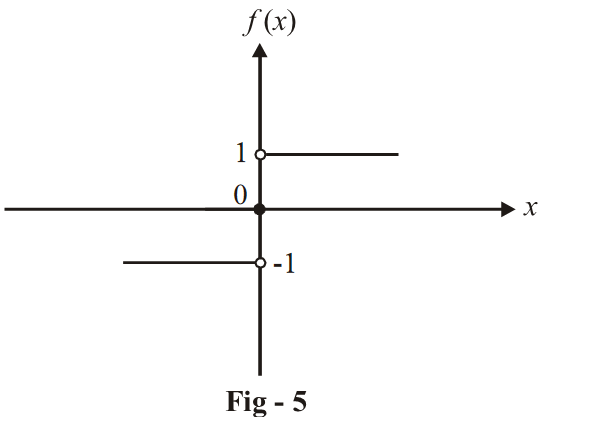At x = 0, we see that

$$LHL = -1,{\rm{ }}RHL{\rm{ }} = 1,f\left( 0 \right){\rm{ }} = {\rm{ }}0$$

$$\Rightarrow {\rm{LHL}} \ne {\rm{RHL}} \ne f\left( 0 \right)$$ and this function is discontinuous.

To summarize, if we intend to evaluate the continuity of a function at x = a, which means that we want to determine whether f (x) will be continuous at x = a or not, we have to evaluate all the three quantities, LHL, RHL and f (a). If these three quantities are finite and equal, f (x) is continuous at x = a. In all other cases, it is discontinuous at x = a

$\boxed{{\text{LHL}}\left( {{\text{at }}x = a} \right) = {\text{RHL}}\left( {{\text{at }}x = a} \right) = f\left( a \right):{\text{ for continuity at }}x = a}$